##### Quantum Physics For Dummies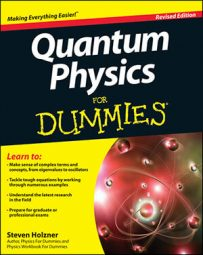Each quantum state of the hydrogen atom is specified with three quantum numbers: n (the principal quantum number), l (the angular momentum quantum number of the electron), and m (the z component of the electron’s angular momentum,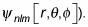How many of these states have the same energy? In other words, what’s the energy degeneracy of the hydrogen atom in terms of the quantum numbers n, l, and m?

Well, the actual energy is just dependent on n, as you see in the following equation: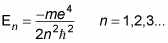That means the E is independent of l and m. So how many states, |n, l, m>, have the same energy for a particular value of n? Well, for a particular value of n, l can range from zero to n – 1. And each l can have different values of m, so the total degeneracy is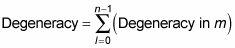The degeneracy in m is the number of states with different values of m that have the same value of l. For any particular value of l, you can have m values of –l, –l + 1, ..., 0, ..., l – 1, l. And that’s (2l + 1) possible m states for a particular value of l. So you can plug in (2l + 1) for the degeneracy in m: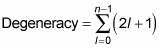And this series works out to be just n2.

So the degeneracy of the energy levels of the hydrogen atom is n2. For example, the ground state, n = 1, has degeneracy = n2 = 1 (which makes sense because l, and therefore m, can only equal zero for this state).

For n = 2, you have a degeneracy of 4: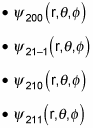Cool.